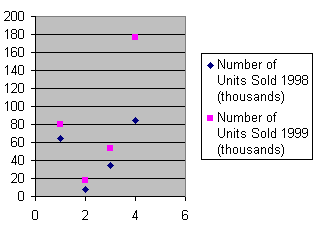# scatter diagram

(redirected from scatter plots)
Also found in: Dictionary, Medical.
Related to scatter plots: Line of best fit

## scatter diagram

(petrology)
(statistics)
A plot of the pairs of values of two variates in rectangular coordinates. Also known as scatter gram.

or

## scattergram

a diagram produced on a graph to illustrate the relationship between two variables by indicating the values of these for each case in the sample. It may be a first stage in data analysis, allowing ‘eye-balling’ of the data to observe any association or CORRELATION between the variables.

## scatter diagram

A graph plotted with dots or some other symbol at each data point. Also called a "scatter plot" or "dot chart."

A Scatter DiagramReferences in periodicals archive ?
Figure 1 shows a scatter plot of quarterly growth rates of real GDP and the GDP deflator, 1949:2 through 1992:4.
Comparing the scatter plots of Examples 7 and 8, we intuitively think that the scatter plot of Example 8 is more concentrated and has a greater tendency of positive correlation than that of the scatter plot of Example 7.
After analyzing several methods of plotting the simulation data, it was discovered that scatter plots provided the best visual representations of the dynamic airflow behavior.
Visualize data using bar charts, line [check] [check] charts, cross table, pie charts, scatter plots and basic table views.
Four appendixes are included: (1) Letter to Principal; (2) Survey to Teachers; (3) Expert Group; and (4) Scatter Plots.
Students can easily view and edit text or numeric data, and plot data as pie charts, pictographs, bar charts, scatter plots, histograms and more.
Results include plots of the regression models, q-q plots, scatter plots, fitted parameters with confidence intervals, input versus estimated data, and goodness of fit statistics.
Bubble plots are XY scatter plots that use symbols to represent not only XY locations, but also a third dimension represented by the size of the symbol.
Now, you can choose from more than 25 types of visualizations like line chart, pie chart, donut chart, area, bar graphs, column charts, scatter plots, stacked series, combination charts, multi-series, etc.
Simple R code produces scatter plots and histograms plus a few fancier things, but nothing approaching the 3-D graphic that my editor obtained (it wasn't me) for the column.

Site: Follow: Share:
Open / Close